# FPGA基础学习(11) -- FIFO设计（style#1）

FIFO是跨时钟域数据传输中常用的缓存器。一般情况下，自己设计的异步FIFO（无特殊说明以下均简称FIFO）虽然能应付90~99%的场景，但是由于设计缺陷，导致在1%的极端情况下会出问题，还不容易发现，所以设计合理的FIFO至关重要。

## 1. 异步指针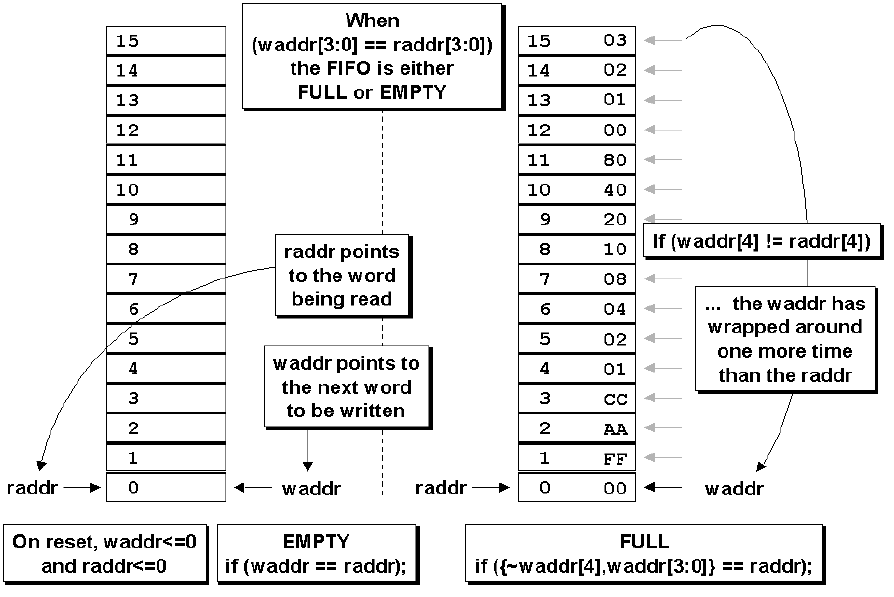• 读指针指向当前要读取的数据位置（空间）
• 写指针指向下一个要写入的位置（空间），即下一个数据来的时候才写入该空间；

## 2. 格雷码计数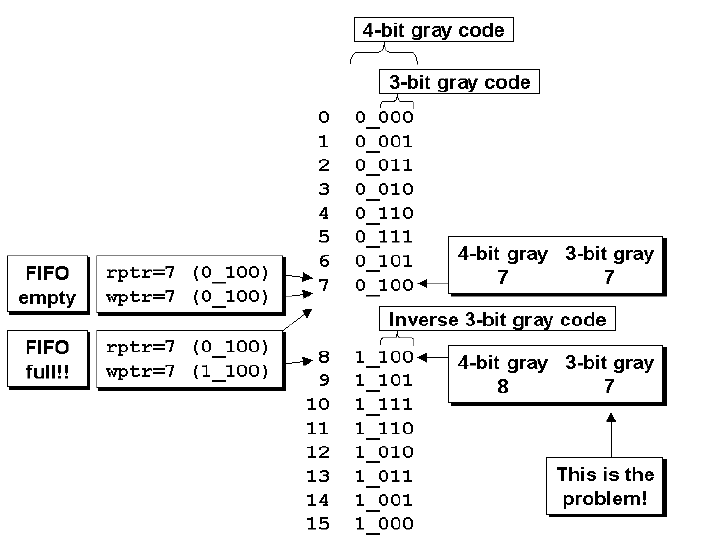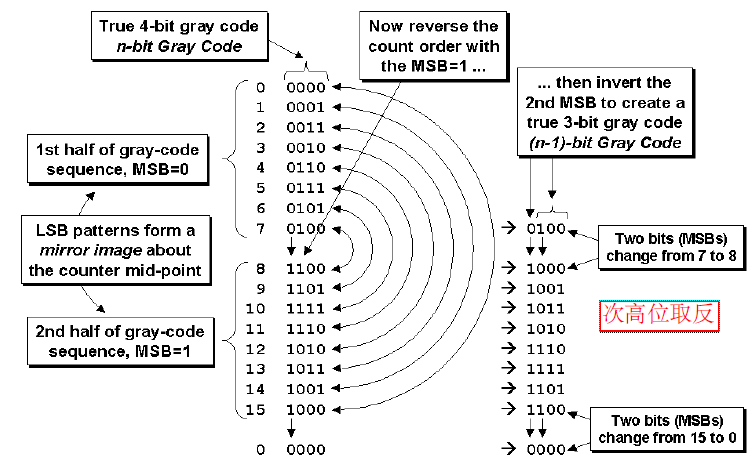• 格雷码各位完全相同，代表空；
• 最高位和次高位不同，其余位相同，代表满

## 3. 如何操作空/满

• “空”：读时钟域中，比较读指针和同步过来的写指针，如果两指针相同 ，为“空”；
• “满”：写时钟域中，比较写时钟和同步过来的读指针，如果两指针最高位和次高位不同，其余位相同，为“满”；

#### - 问题1：同步格雷码的过程中变化了2次，但只有一次被对方时钟域采集到，会不会造成多位同步出错的问题？

（ps：实际上对原位的问题和解答翻译理解不到位，此处只能我的理解简单说一下）

#### - 问题2：假设写时钟频率更高，写指针在与读指针比较的时候，还没来得及产生满信号，写这一头还在不停的写入数据，会不会造成上溢出？（读空是同样的道理）

（ps：这个问题是FIFO操作最常见的问题）

## 4. 仿真结果


module fifo1_sim();
parameter DSIZE = 8;
parameter ASIZE = 3;

wire  [DSIZE-1:0] rdata;
wire              wfull;
wire              rempty;

reg   [DSIZE-1:0] wdata;
reg   winc, wclk, wrst_n;
reg   rinc, rclk, rrst_n;

reg             wr_en;
reg             rd_en;
reg             wr_rd;

initial begin
wrst_n = 1'b0;
rrst_n = 1'b0;
#50;
wrst_n = 1'b1;
rrst_n = 1'b1;
end

initial begin
wclk = 1'b0;
#10;
forever #5 wclk =~wclk;
end

initial begin
rclk = 1'b0;
#10;
forever #10 rclk =~rclk;
end

initial begin
wr_en = 1'b0;
rd_en = 1'b0;
wr_rd = 1'b0;
#100;
wr_en = 1'b1;
rd_en = 1'b0;
wr_rd = 1'b0;
#150
wr_en = 1'b0;
rd_en = 1'b1;
wr_rd = 1'b0;
#200
wr_en = 1'b0;
rd_en = 1'b0;
wr_rd = 1'b1;
#200
wr_en = 1'b0;
rd_en = 1'b0;
wr_rd = 1'b0;

end

always @(posedge wclk or negedge wrst_n) begin
if(wrst_n == 1'b0) begin
wdata <= 8'h10;
winc <= 1'b0;
end else if(wr_en) begin
wdata <= wdata + 8'd1;
winc <= 1'b1;
end else if(wr_rd) begin
wdata <= wdata + 8'd1;
winc <= 1'b1;
end else begin
wdata <= wdata;
winc <= 1'b0;
end
end

always @(posedge rclk or negedge rrst_n) begin
if(rrst_n == 1'b0) begin
rinc <= 1'b0;
end else if(rd_en) begin
rinc <= 1'b1;
end else if(wr_rd) begin
rinc <= 1'b1;
end else begin
rinc <= 1'b0;
end
end

fifo1 #( DSIZE,ASIZE)
fifo1_i
(
.rdata  (rdata),
.wfull  (wfull),
.rempty (rempty),

.wdata  (wdata),
.winc   (winc),
.wclk   (wclk),
.wrst_n (wrst_n),
.rinc   (rinc),
.rclk   (rclk),
.rrst_n (rrst_n)
);

endmodule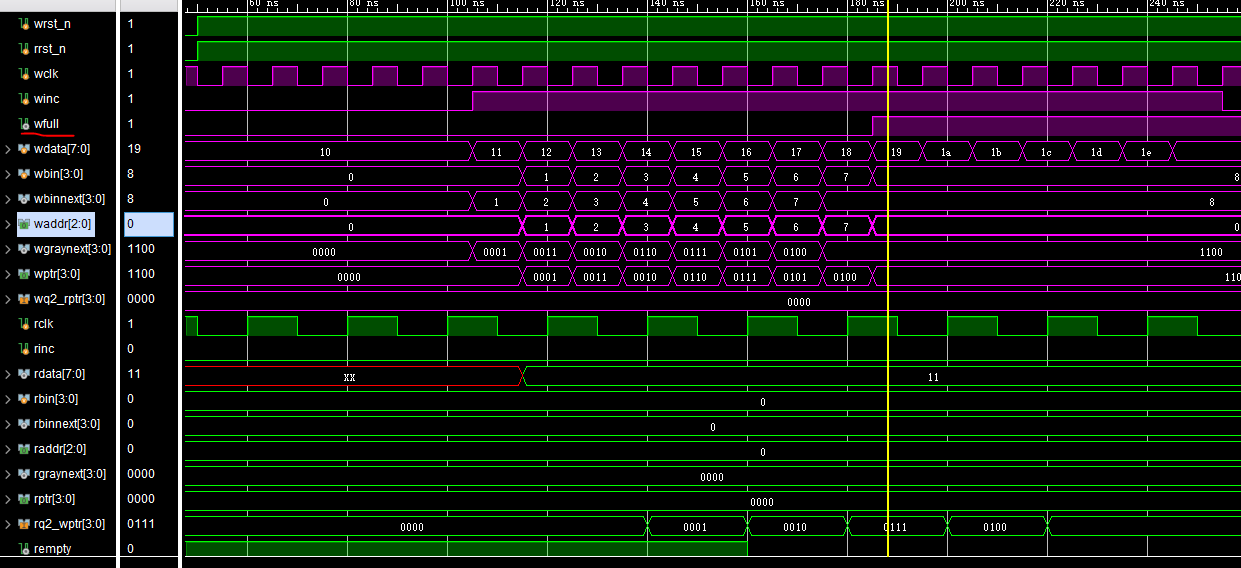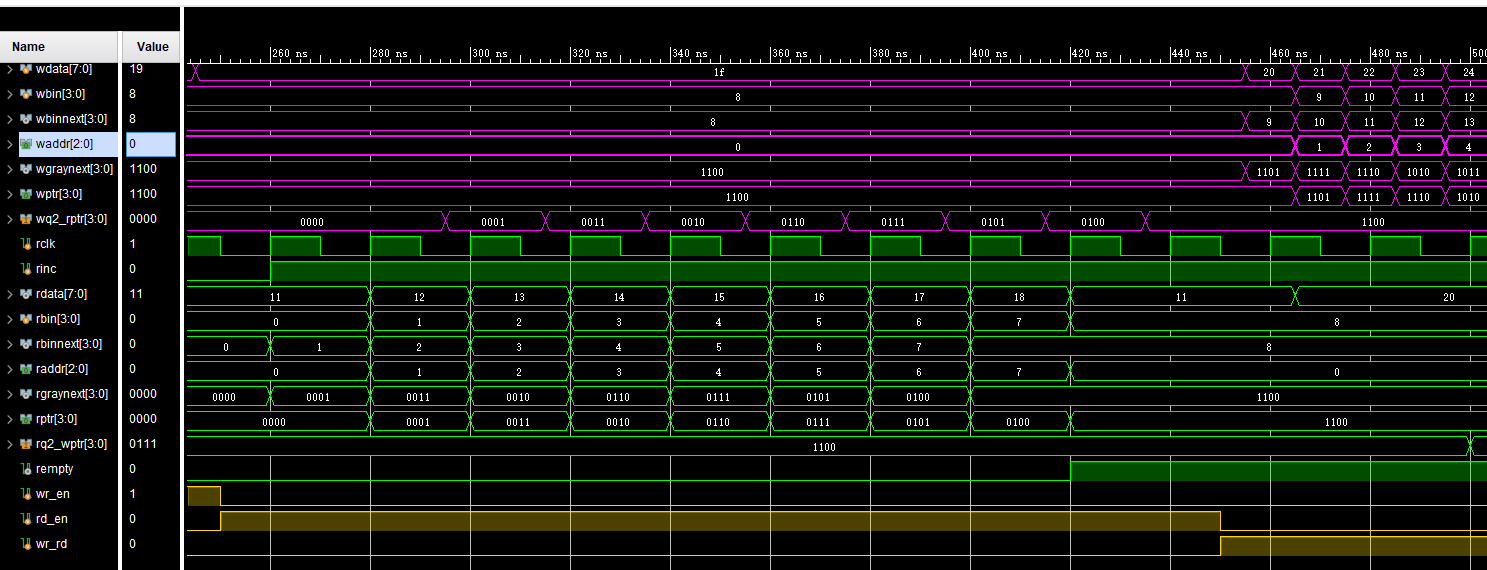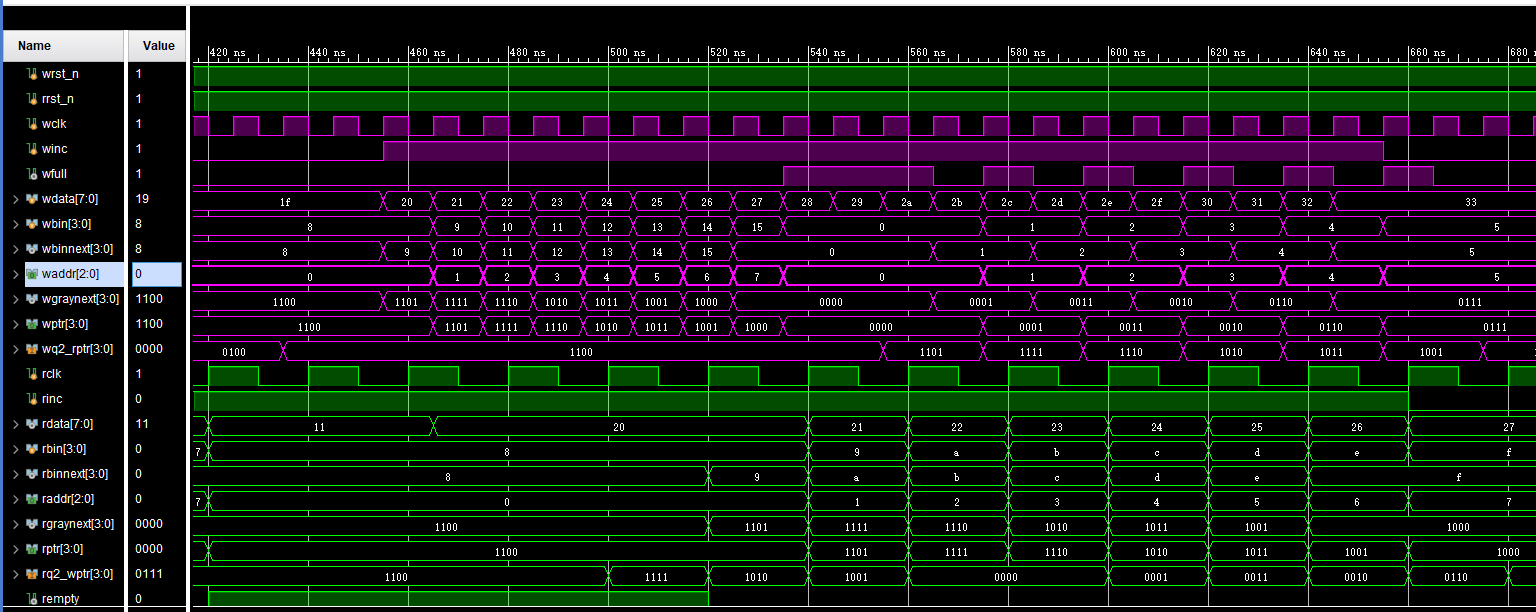## 5. 特别说明

• 原文有很多细节描述，实际上并不是理解很透彻。如问题1，还有比较二进制编码和格雷码优劣的论述。
• 该篇论文（V1.2）感觉有问题，源代码实现的应该是style#2的框图，如下图所示，即二进制编码驱动RAM的地址，格雷码进行指针比较。多说一句，源码中格雷码和RAM的地址是一一对应的标识关系，格雷码不是地址，只是对地址空间的一个描述，用于跨时钟域比较。
• 网上有帖子争论较大的是，要是读写时钟频率差距过大，比较1000倍，FIFO会不会出问题？从论文的角度分析，不会出问题（可以展开讨论）。从而牵扯另外的一个话题，FIFO最小深度的计算。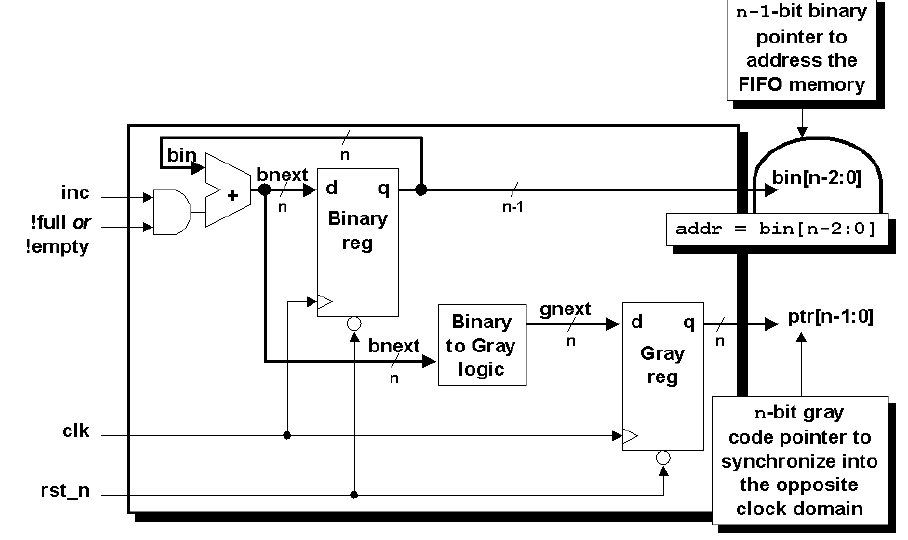posted @ 2020-03-04 14:03  肉娃娃  阅读(1246)  评论(0编辑  收藏  举报